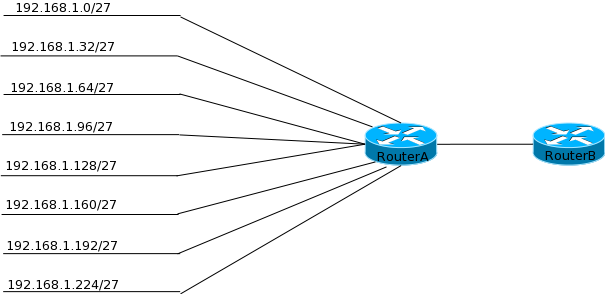You already know from the previous chapter that routers function by creating a table of all networks it knows about. This table is called the routing table and routers use routing protocols to tell each other about the networks they know of. As networks increase, so do the number of entries in a routing table. Large routing tables cause increased processing and lower response time in a router. To reduce the size of routing tables, networks can be grouped together or summarized using a mask that incorporates them all.  For example, in figure 2-5, a 192.168.10.0/24 subnet has been divided into smaller subnets of /27 mask. All of these networks connect to RouterA which it turn is advertising these routes to RouterB. Without summarization, RouterB will come to know of 8 networks which are available via RouterA. Since these networks are contagious subnets can have been subnetted from a /24 address, they can be summarized back into 192.168.1.0/24 network by RouterA while advertising to RouterB. This way, RouterB comes to know of one large /24 network only instead of 8 smaller /27 networks.

Figure 2-5 SummarizationSummarization is similar to VLSM but in the opposite direction. When using VLSM you move to the right in terms of the bits (/24 to /25, /25 to /26, so on and so forth) while during summarization you move to the left (example /27 to /24).

Summarization is somewhat simple if you remember the following:

1. You can only summarize in the block sizes you learned about in VLSM – 128,64,32,16,8,4.
2. The network address used for the summarized address is the first network address in the block.

For example, if you want to summarize networks 192.168.8.0 through 192.168.15.0, first find the block size you can use. There are 8 networks so the block size of 8 can be used. The first network address in the block is 192.168.8.0. Now to find the mask of the summarized route, remember the mask used for a block of 8 – 248. You can also deduct the block size from 256 to find the mask. Since we are summarizing the third octet the subnet mask for the summary address will be 255.255.248.0.

Take another example, 172.16.0.0 through 172.16.35.0. This one is not as simple as the first one. Notice that you have 36 networks to summarize which does not conform to the block sizes. There are two things that you can do here:

1. Summarize in block size of 32 (mask of 224). This will give you a summary address of 172.16.0.0 255.255.224.0 but will only summarize networks 172.16.0.0 through 172.16.31.0. The rest of the 4 networks will be advertised as individual routes.
2. Summarize in block of 64 (mask of 192). This will give you a summary address of 172.16.0.0 255.255.192.0 but will summarize networks 172.16.0.0 through 172.16.63.0.

The correct answer depends on the network. If you are planning to add networks 36 to 63 then the second options works. Otherwise the first option is the best one.

Take a third example where you know the summary address of 172.10.16.0 with a mask of 255.255.224.0 and need to find which networks are being summarized. This is really easy. The third octet is the interesting octet and gives a block size of 32. This means the networks 172.10.16.0 through 172.10.47.0 have been summarized.

As a final example, consider the following networks:

• 192.168.1.0/25
• 192.168.1.128/25
• 192.168.2.0/24
• 192.168.3.0/24
• 192.168.4.0/26
• 192.168.4.64/26
• 192.168.4.128/26
• 192.168.4.192/26

Try to figure out the summary address that can be used for these networks. If you look carefully the third octet forms a contiguous block of 4 and can be summarized with the address 192.168.1.0 255.255.252.0 or 192.168.1.0/22.

In the last example notice that we summarized a contiguous block of class C using a mask. This is called supernetting. Supernetting is an extension of VLSM and summarization. In summarization you summarize networks subnetted while in supernetting you summarize a block of contiguous blocks of Class A, B or C networks. Supernetting is usually practiced by ISPs to reduce the Internet routing table size.